# AP Chemistry Practice Test 44

### Test Information13 questions19 minutes

`Questions 1-3 refer to the following information.Use the information on the following proposed mechanism to answer questions.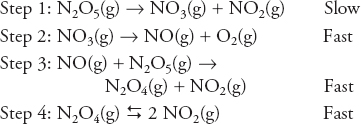`

1. The above represents a proposed mechanism for the gaseous dinitrogen pentoxide, N2O5. What are the overall products of the reaction?

2. Choose the energy profile that best describes this mechanism.

3. What is the rate law for the reaction?

`Questions 4-6 refer to the following information.Use the information on the containers in the following diagram to answer questions.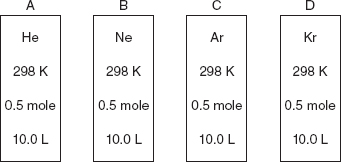Approximate molar masses: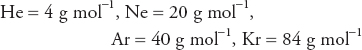`

4. If a sample of Kr effuses at a rate of 35 mL per second at 298 K, which of the gases below will effuse at approximately double the rate under the same conditions?

5. Assuming all four gases are behaving ideally, which of the following is the same for all the gas samples?

6. Which of the gases will show the greatest deviation from ideal behavior?

`Questions 7-10 refer to the following information.Use the information on the containers in the following diagram to answer questions concerning the following equilibrium.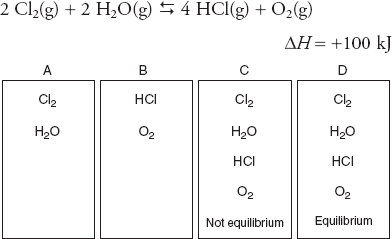`

7. An equilibrium mixture (container D) is at 75°C. Which of the following changes may increase the amount of the HCl in the container?

8. Containers A, B, and C are not at equilibrium. If each container begins with equal amounts of the indicated substances present at the same temperature, which of the three will reach equilibrium first?

9. If the initial partial pressure of Cl2 in container A is 1.0 atm and the initial partial pressure of H2O is also 1.0 atm, what will be the pressure at equilibrium?

10. If the moles of HCl in container B are equal to five times the O2 present, what can be said about the moles of O2 present at equilibrium?

`Questions 11-13 refer to the following information.Use the following information on the bases in the following diagram to answer questions.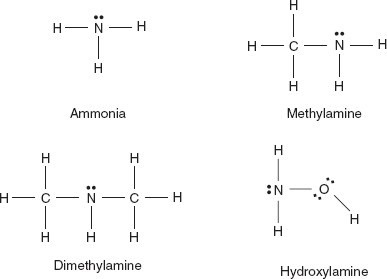Ammonia is only present as a reference. Questions only refer to the other three bases.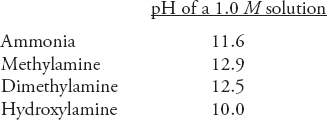`

11. Solutions of methylamine, dimethylamine, and hydroxylamine are titrated. The base concentrations were 0.1000 M, and 0.1000 M hydrochloric acid, HCl, was used for the titrations. Which of the three bases will yield the highest pH at the equivalence point?

12. All the bases in the diagram behave as Brønsted-Lowry bases in the same way; in each case, they accept a hydrogen ion to the same atom. How is this acceptance of a hydrogen ion accomplished?

13. Which of the following explains why the pH of a hydroxylamine solution is lower than any of the other solutions?